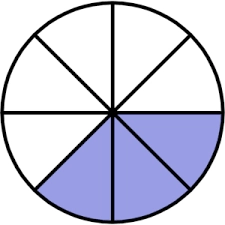# Negative fractions

I am a number that is equal to -3/4 subtracted from the sum of 3/5 and -1/3. What number am I?

x =  61/60 = 1 160

### Step-by-step explanation:Did you find an error or inaccuracy? Feel free to write us. Thank you!

Tips for related online calculators
Need help calculating sum, simplifying, or multiplying fractions? Try our fraction calculator.